All You Need to Know About

# VLOOKUP Formula in Excel

The Excel VLOOKUP Formula is one of the most used Excel Formulas. Learn about the different ways you can apply the VLOOKUP Function in Excel!

Here are the top things on what you can do with the VLOOKUP Formula in Excel:

## VLOOKUP Function: Introduction

What does it do?

Searches for a value in the first column of a table array and returns a value in the same row from another column (to the right) in the table array.

Formula breakdown:

=VLOOKUP(lookup_value, table_array, col_index_num, [range_lookup])

What it means:

=VLOOKUP(this value, in this list, and get me value in this column, Exact Match/FALSE/0])

Excel`s VLOOKUP function is arguably the most used function in Excel but can also be the most tricky one to understand.  I will show you a VLOOKUP example and in a few steps you will be able to extract values from a table and use them to do your custom reports and analysis.

You will be using VLOOKUP with confidence after this tutorial!

STEP 1: We need to enter the VLOOKUP function in a blank cell:

## =VLOOKUP(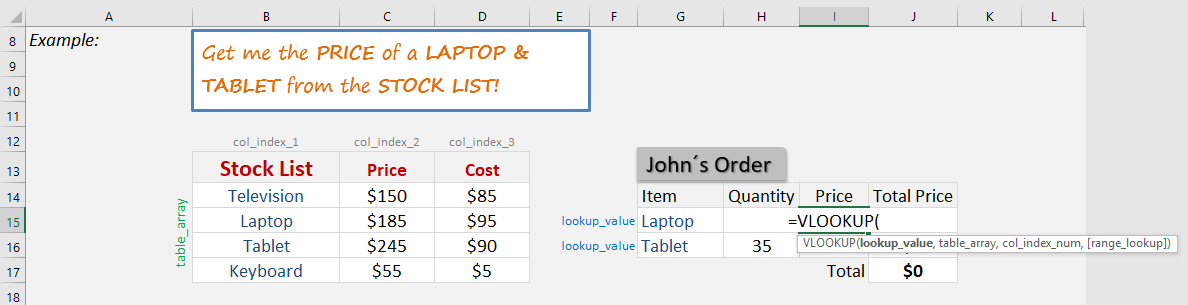STEP 2: The VLOOKUP arguments:

## Lookup_value

What is the value that you want to look for?

In our first example, it will be Laptop, so select the Item name

## =VLOOKUP(G15,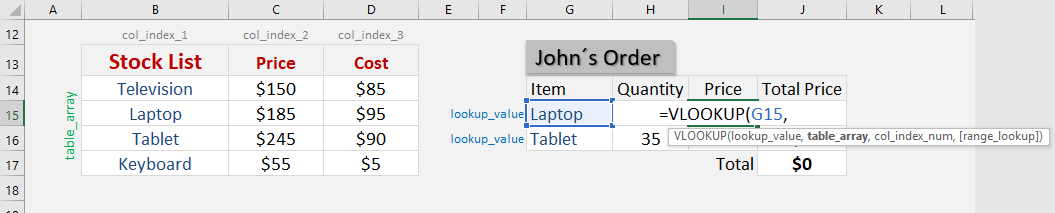## Table_array

What is the table or range that contains your data?

Make sure to select the stock list table so that our VLOOKUP formula will search here

## =VLOOKUP(G15, B14:D17,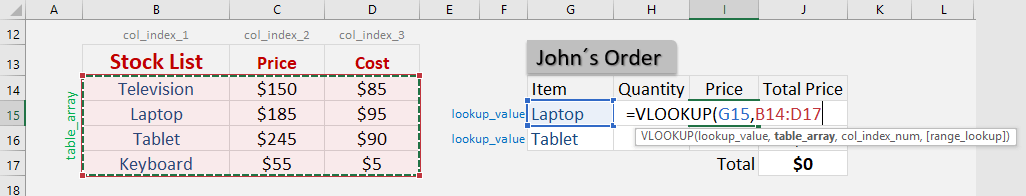Ensure that you press F4 so that you can lock the table range.

## =VLOOKUP(G15, \$B\$14:\$D\$17,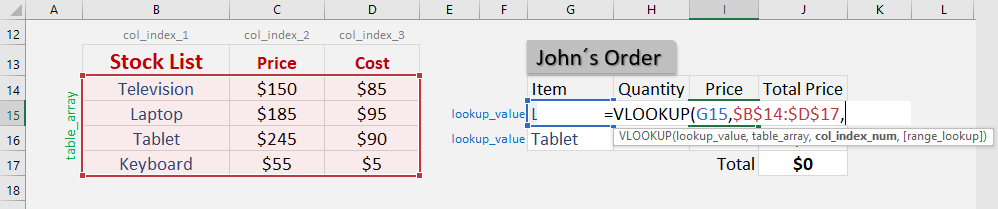## Col_index_num

What is the column that you want to retrieve the value from?

Since we want to get the price, our price is on the 2nd column of our source data

## =VLOOKUP(G15, \$B\$14:\$D\$17, 2,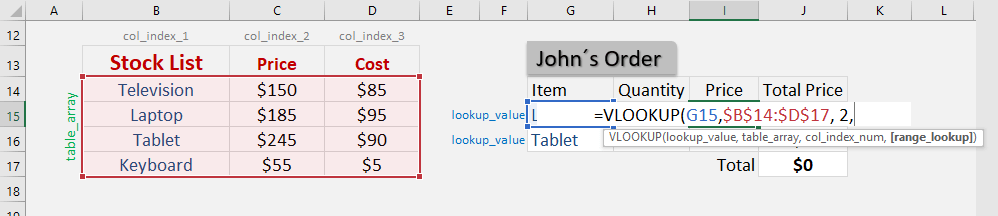## Range_lookup

What kind of matching do you need?

We want an exact match of the Laptop text so make sure FALSE is selected.

## =VLOOKUP(G15, \$B\$14:\$D\$17, 2, FALSE)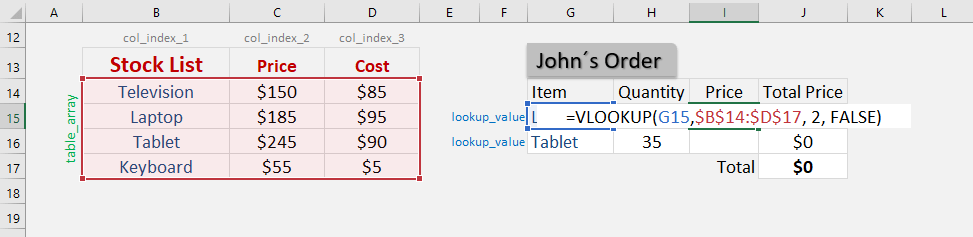Apply the same formula to the rest of the cells by dragging the lower right corner downwards.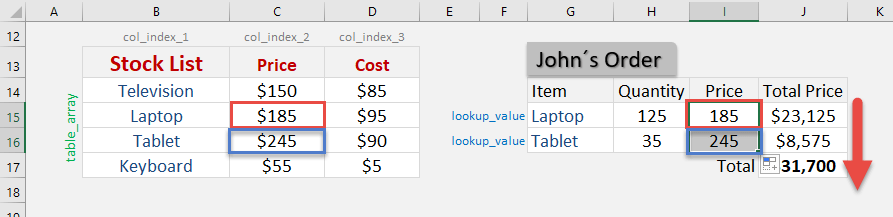You now have all of the results!

## Vlookup in Multiple Excel Sheets

What does it do?

Searches for a value in the first column of a table array and returns a value in the same row from another sheet´s column (to the right) in the table array.

Formula breakdown:

=VLOOKUP(lookup_value, table_array, col_index_num, [range_lookup])

What it means:

=VLOOKUP(this value in Sheet1, in this list in Sheet2, and get me value in this column in Sheet2, Exact Match/FALSE/0])

Sometimes you are faced with a situation where you have a list of data and you want to bring in complimentary data from a different sheet within the same workbook.

Let’s say that you have a list of “items” in a table within Sheet1 and you want to bring  in their corresponding “item id’s” from Sheet2.

You can manually copy and paste the item id’s from Sheet2 to Sheet1 but that would take too long and you are also prone to errors.

The quick and error-proof way is by using the VLOOKUP function.

See the tutorial below of how this can be achieved.

## Named Ranges with Vlookup Formula

What does it do?

Searches for a value in the first column of a table array and returns a value in the same row from another column (to the right) in the table array.

Formula breakdown:

=VLOOKUP(lookup_value, table_array, col_index_num, [range_lookup])

What it means:

=VLOOKUP(this value, in this Named Range, and get me value in this column, Exact Match/FALSE/0])

A Named Range makes it easier to understand Excel formulas, especially if the said formula contains an array argument.

A Named Range can be a cell, a cell range, a Table, a function or a constant.

STEP 1: To define a Named Range in Excel you need to select the cell/cell range/Table/function/constant and go to the Name Box which is located on the top left hand corner of the workbook – next to the Formula Bar.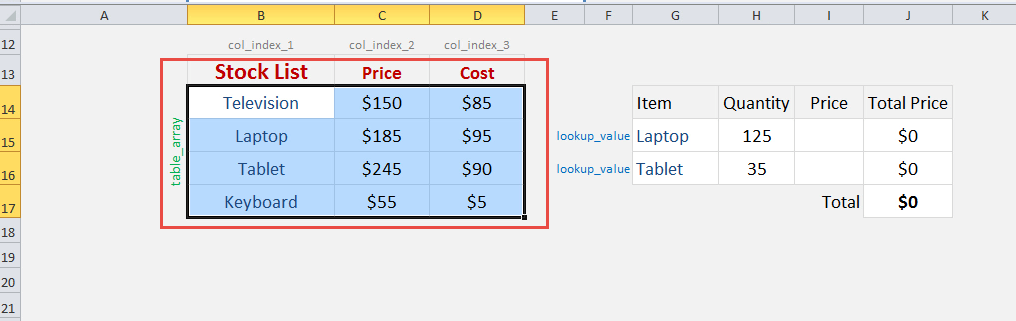STEP 2: In here you can name your range whatever you like (make sure there are no spaces) and press Enter.  You can view your Named Range by clicking on the drop down box in the Name Box.  In our example we will give this a name of StockList.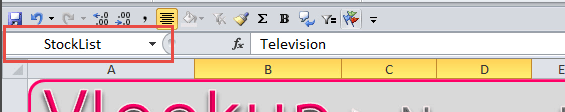You can also view/edit/delete your Named Range by going to the Formulas tab in the Ribbon menu and selecting Name Manager.

STEP 3: Now that you are all set, each time you are creating a formula, like a Vlookup formula, it is best to use a Named Range as it makes the formula easier to understand and maintain.

We need to enter the Vlookup function:

## =VLOOKUP(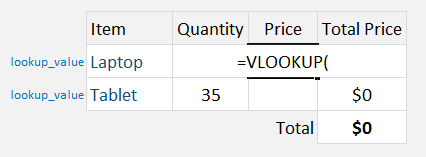The Vlookup arguments:

## lookup_value

What are we looking for?

Reference the cell that contains the text or value:

## =VLOOKUP(G15,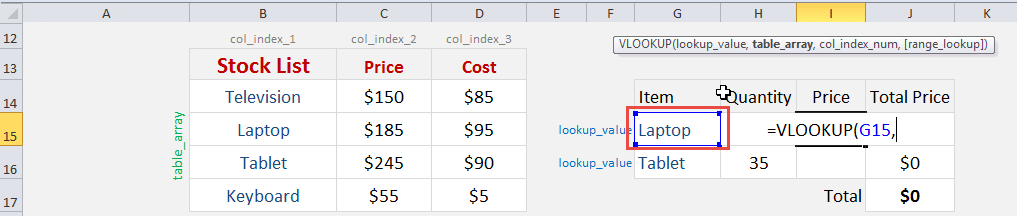## table_array

From which list are we doing a lookup on?

Place in the Named Range of the Stock List:

## =VLOOKUP(G15, StockList,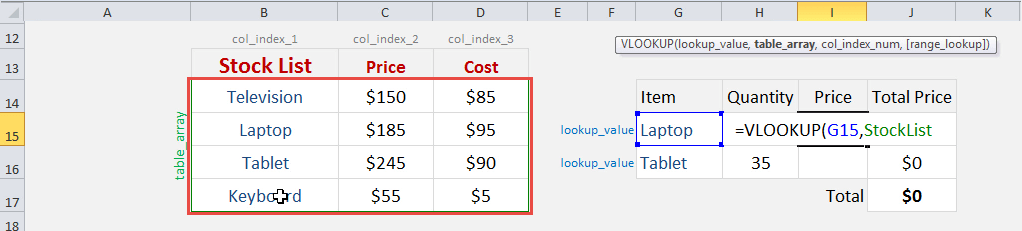## col_index_num

From which column do we want to retrieve the value?

We want to retrieve the Price which is the SECOND column from our table array:

## =VLOOKUP(G15, StockList, 2,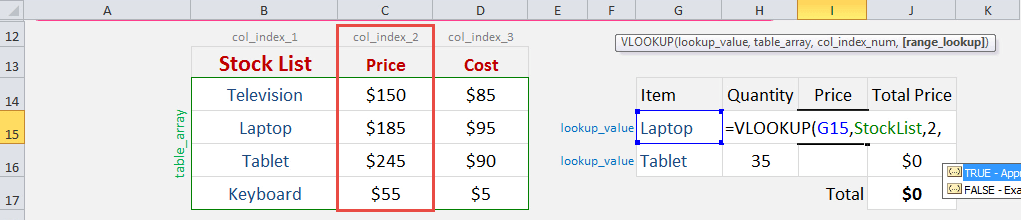## [range_lookup]

Do we want an exact match?

Place in FALSE to signify that we want an exact match:

## =VLOOKUP(G15, StockList, 2, FALSE)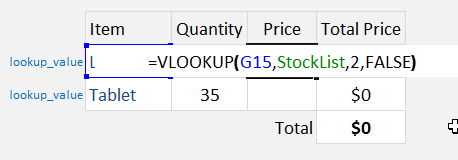The price now dynamically changes based on your selection:

Latest Tutorials

March 5, 2021

March 1, 2021

## VLOOKUP Example: Vlookup with a Drop Down List

February 26, 2021

August 6, 2020

October 31, 2018

June 7, 2016